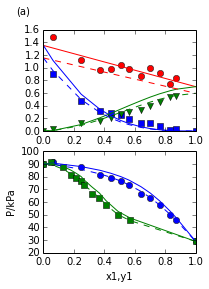# Chapter 12 : Solution Thermodynamics Applications¶

## Example 12.1 page no : 196¶

In :
%matplotlib inline
from matplotlib.pyplot import plot,suptitle,xlabel,ylabel,subplot
from numpy import zeros,array,exp,round
import math

# Variables
P = array([90.15,91.78,88.01,81.67,78.89,76.82,73.39,66.45,62.95,57.70,50.16,45.70,29.00]);
x1 = array([0.000,0.063,0.248,0.372,0.443,0.508,0.561,0.640,0.702,0.763,0.834,0.874,1.000]);
y1 = array([0.000,0.049,0.131,0.182,0.215,0.248,0.268,0.316,0.368,0.412,0.490,0.570,1.000]);
x2 = 1-x1;
y2 = 1-y1;
P1_sat = P
P2_sat = P
K = zeros(13);
ln_V1 = zeros(13)
ln_V2 = zeros(13)
# Calculations and Results
for i in range(13):
if(i !=  0):
ln_V1[i] = round(math.log(y1[i]*P[i]/(x1[i]*P1_sat)),3);
if(i !=  12):
ln_V2[i] = round(math.log(y2[i]*P[i]/(x2[i]*P2_sat)),3);

ln_V1 = None
ln_V2 = None
k = zeros(13)
for i in range(1,13):
K[i] = round(((x1[i]*ln_V1[i])+(x2[i]*ln_V2[i]))/(x1[i]*x2[i]),3);  			#K = G_E/(x1*x2*R*T)
k[i] = round(((x1[i]*ln_V1[i])+(x2[i]*ln_V2[i])),3);  			#K = G_E/(R*T)

K = None
k = None
K = None
k = None
A21 = 0.70;
A12 = 1.35;
K_new = round((A21*x1)+(A12*x2),3);
#Using Eqn (12.10(a) and 12.10(b))
ln_V1_new = round((x2*x2)*(A12+(2*(A21-A12)*x1)),3);
V1_new = round(exp(ln_V1_new),3);
ln_V2_new = round((x1*x1)*(A21+(2*(A12-A21)*x2)),3);
V2_new = round(exp(ln_V2_new),3);
#Using Eqn (12.11)
P_new = (x1*V1_new*P1_sat)+(x2*V2_new*P2_sat);

A21_new = 0.596;
A12_new = 1.153;

K_new1 = round((A21_new*x1)+(A12_new*x2),3);
#Umath.sing Eqn (12.10(a) and 12.10(b))
ln_V1_new1 = round((x2*x2)*(A12_new+(2*(A21_new-A12_new)*x1)),3);
V1_new1 = round(exp(ln_V1_new1),3);
ln_V2_new1 = round((x1*x1)*(A21_new+(2*(A12_new-A21_new)*x2)),3);
V2_new1 = round(exp(ln_V2_new1),3);
#Umath.sing Eqn (12.11)
P_new1 = (x1*V1_new1*P1_sat)+(x2*V2_new1*P2_sat);

subplot(220)
plot(x1,P,'bo')
plot(y1,P,'gs')

plot(x1,P_new,'b-')
plot(y1,P_new,'g-')

plot(x1,P_new1,'b--')
plot(y1,P_new1,'g--')

suptitle('(a)')
xlabel('x1,y1')
ylabel('P/kPa')

subplot(222)
plot(x1,ln_V1,'bs')
plot(x1,ln_V2,'gv')

plot(x1,K,'ro')

plot(x1,K_new,'r-')
plot(x1,ln_V1_new,'b-')
plot(x1,ln_V2_new,'g-')

plot(x1,K_new1,'r--')
plot(x1,ln_V1_new1,'b--')
plot(x1,ln_V2_new1,'g--')

Out:
[<matplotlib.lines.Line2D at 0x10fccef90>]## Example 12.2 page no : 198¶

In :
from numpy import poly1d

#H_E = x1x2(40x1+20x2)   (A)
#Find H1_E and H2_E

#H1_E = H_E+((1-x1)*(dH_E/dx1))  let d = dH_E/dx1

#H2_E = H_E-(x1*(dH_E/dx1))  let d = dH_E/dx1

#Replacing x2 = 1-x1 in (A)

# Calculations
H_E = poly1d([0, 20, 0, -20],'x1','c');
d = poly1d([20 ,0 ,-60],'x1','c');
H1_E = poly1d([20, 0, -60, 40],'x1','c');
H2_E = poly1d([0 ,0 ,0 ,40],'x1','c');

# Results
print 'Expression For H1_E(x1) is ',H1_E
print 'Expression For H2_E(x1) is ',H2_E

Expression For H1_E(x1) is     4        2
1 c - 2800 c + 4.8e+04 c
Expression For H2_E(x1) is     4      3
1 c - 40 c


## Example 12.4 page no : 201¶

In :
# Program to Find the Heat of Formation of LiCl

#          Li + 0.5Cl2 --> LiCL(s)       (A)

#   LiCl(s) + 12H2O(l) --> LiCl(12H2O)   (B)

#Net Reaction
#Li + 0.5Cl2 +12H2O(l) --> LiCl(12H2O)   (Net)

# Variables
#From Table C.4
Hf_A = -408610;			#[J]
Hf_B = -33614;			#[J]

# Calculations
Hf_Net = Hf_A+Hf_B;			#[J]

# Results
print 'Heat of formation of LiCl in 12mol H2O at 298.15K is',Hf_Net,'J'

Heat of formation of LiCl in 12mol H2O at 298.15K is -442224 J


## Example 12.5 page no : 204¶

In :
import math

# Variables
M_LiCl = 42.39;
M_H2O = 18.015;
T1 = 298.15;			#[K]
T2 = 405.15;			#[K]

# Calculations and Results
#Step a
m_LiCl = 0.15*2;
m_H2O = 2-m_LiCl;
n_LiCl = (m_LiCl*1000)/M_LiCl
n_H2O = (m_H2O*1000)/M_H2O;
dH_LiCl = -33800;
dH_a = -n_LiCl*dH_LiCl			#[J/s]

#Step b
m_LiCl = 0.15*2;
m_H2O = 0.45;
n_LiCl = (m_LiCl*1000)/M_LiCl;
n_H2O = (m_H2O*1000)/M_H2O;
dH_LiCl = -23260;
dH_b = n_LiCl*dH_LiCl			#[J/s]

#Step c
m_LiCl = 0.75;
Cp = 2.72;
dT = T2-T1;
dH_c = m_LiCl*Cp*dT*1000			#[J/s]

#step d
m_H2O = 2-m_LiCl;
dH_T2 = 2740.3;			#[KJ/s/mol] form Steam Tables
dH_T1 = 104.8;			#[KJ/s/mol]  from Steam Tables
dH_d = m_H2O*(dH_T2-dH_T1)*1000			#[J/s]

dH = round((dH_a+dH_b+dH_c+dH_d)/1000,1);

print 'The required Heat Transfer rate is ',dH,'kW or KJ/s'

The required Heat Transfer rate is  3587.2 kW or KJ/s


## Example 12.6 page no : 207¶

In :
# Variables
T0 = 298.15;			#[K]
T = 361.5;			#[K]
mT = 1.25;			#[Kg/s]  10% NaOH
m_steam = 1;			#[Kg/s] at P = 76 torr and 361.5K
m_50NaOH = mT-m_steam;			#[Kg/s]  at 361.5K

# Calculations
#From Steam tables
#at 76 torr and 361.15K
H_steam = 2666;			#[KJ/kg]
#for 10% NaOH soln at 294.15K
H_10NaOH = 79;			#[KJ/Kg]
#for 50% NaOH soln at 361.15K
H_50NaOH = 500;			#[KJ/Kg]

dH = (m_steam*H_steam)+(m_50NaOH*H_50NaOH)-(mT*H_10NaOH);
Q = dH;

# Results
print 'Heat Transfer rate',Q,'kW or kJ/s'

Heat Transfer rate 2692.25 kW or kJ/s


## Example 12.9 page no : 208¶

In :
# Variables
T = 294.15;		                	        #[K]
m_NaOH_soln = 1.;		            	    #[kg]
m_NaOH_solid = 0.45*m_NaOH_soln;			#[Kg]
m_H2O = 0.55*m_NaOH_soln;			#[Kg]

#From Steam Tables
H_NaOH_soln = 216.;			#[kJ/Kg]
H_NaOH_solid = 1113.;			#[kJ/Kg]
H_H2O = 88.;			#[kJ/Kg]

# Calculations
dH = m_NaOH_soln*H_NaOH_soln-(m_NaOH_solid*H_NaOH_solid)-(m_H2O*H_H2O);
Q = dH;

# Results
print 'Heat Transferred per Kg of NaOH Soln',Q,'kW or kJ/kg'

Heat Transferred per Kg of NaOH Soln -333.25 kW or kJ/kg